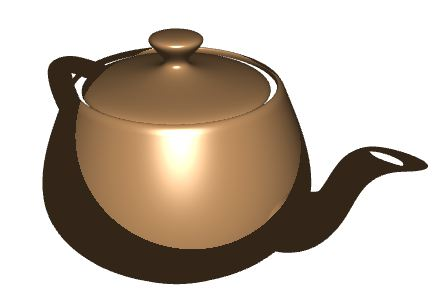Prev: Point Lights Next: Texture Coordinates

Spotlights are restricted point lights, i.e.  the light rays are only emitted in a restricted set of directions. Commonly we use a cone to define this restriction, but other shapes are possible.

The following diagram shows a cone shaped spotlight and the relevant data.• position: this is the cone’s apex (sp);
• spot direction: the vector that defines the direction of the axis of the cone (sd);
• cutoff angle: the aperture of the cone. In here we are going to assume that the angle is measured from the direction vector to the border of the cone ($\alpha$).

The vertex shader is almost the same as for point lights. Just copy the light block in the fragment shader below to the vertex shader and it should work nicely.

It is up to the fragment shader to determine if a fragment is inside the cone, i.e. the dot product between the light’s direction and the spot’s direction is less than some cutoff value, and lit it accordingly. Actually, instead of using an angle, we should provide the cosine of the angle to avoid having to compute the inverse cosine in a shader.

#version 330

out vec4 colorOut;

layout (std140) uniform Materials {
vec4 diffuse;
vec4 ambient;
vec4 specular;
float shininess;
};

layout (std140) uniform Lights {
vec4 l_pos, l_spotDir;
float l_spotCutOff;
};

in Data {
vec3 normal;
vec3 eye;
vec3 lightDir;
} DataIn;

void main() {

float intensity = 0.0;
vec4 spec = vec4(0.0);

vec3 ld = normalize(DataIn.lightDir);
vec3 sd = normalize(vec3(-l_spotDir));

// inside the cone?
if (dot(sd,ld) > l_spotCutOff) {

vec3 n = normalize(DataIn.normal);
intensity = max(dot(n,ld), 0.0);

if (intensity > 0.0) {
vec3 eye = normalize(DataIn.eye);
vec3 h = normalize(ld + eye);
float intSpec = max(dot(h,n), 0.0);
spec = specular * pow(intSpec, shininess);
}
}

colorOut = max(intensity * diffuse + spec, ambient);
}

An example image is presented below.Prev: Point Lights Next: Texture Coordinates

### 2 Responses to “GLSL Tutorial – Spotlights”

1.I’ve been following your tutorial and for some reason the vertex from the point lights tutorial doesn’t seem to work with this fragment Shader.

I get this error:

Block “Lights” has different definition accross different shaders.

Any suggestions? My code is the same as the given tutorial’s.

•Hi,

That is a bug in the tutorial, the vertex shader is not exactly the same as the point light version. To get it working you must copy the Light block definition from the fragment shader to the vertex shader.

This site uses Akismet to reduce spam. Learn how your comment data is processed.